# Mean State

Period Mean (original grids) [W/m2]
Model Period Mean (intersection) [W/m2]
Model Period Mean (complement) [W/m2]
Benchmark Period Mean (intersection) [W/m2]
Benchmark Period Mean (complement) [W/m2]
Bias [W/m2]
RMSE [W/m2]
Phase Shift [months]
Bias Score 
RMSE Score 
Seasonal Cycle Score 
Spatial Distribution Score 
Interannual Variability Score 
Overall Score 
Benchmark [-] -56.1
CRUNCEPv7 [-] -53.7 -53.4 -56.5 -49.8 3.55 14.7 2.47 0.529 0.420 0.600 0.935 0.504 0.568
GSWP3v1 [-] -63.4 -63.2 -56.5 -49.8 -6.77 14.9 1.90 0.549 0.417 0.725 0.993 0.726 0.638
WFDEI [-] -43.3 -42.9 -56.5 -49.8 13.6 21.8 2.90 0.340 0.370 0.515 0.835 0.688 0.520
Period Mean (original grids) [W/m2]
Model Period Mean (intersection) [W/m2]
Model Period Mean (complement) [W/m2]
Benchmark Period Mean (intersection) [W/m2]
Benchmark Period Mean (complement) [W/m2]
Bias [W/m2]
RMSE [W/m2]
Phase Shift [months]
Bias Score 
RMSE Score 
Seasonal Cycle Score 
Spatial Distribution Score 
Interannual Variability Score 
Overall Score 
Benchmark [-] -75.5
CRUNCEPv7 [-] -83.3 -84.1 -75.8 -54.3 -7.88 18.3 0.372 0.718 0.570 0.963 0.996 0.516 0.722
GSWP3v1 [-] -91.8 -92.5 -75.8 -54.3 -16.4 22.7 0.315 0.557 0.572 0.969 0.990 0.696 0.726
WFDEI [-] -74.0 -74.9 -75.8 -54.3 1.08 19.7 0.739 0.744 0.522 0.911 0.962 0.729 0.732
Period Mean (original grids) [W/m2]
Model Period Mean (intersection) [W/m2]
Model Period Mean (complement) [W/m2]
Benchmark Period Mean (intersection) [W/m2]
Benchmark Period Mean (complement) [W/m2]
Bias [W/m2]
RMSE [W/m2]
Phase Shift [months]
Bias Score 
RMSE Score 
Seasonal Cycle Score 
Spatial Distribution Score 
Interannual Variability Score 
Overall Score 
Benchmark [-] -76.3
CRUNCEPv7 [-] -77.2 -77.3 -77.0 -61.8 1.36 16.2 1.46 0.707 0.515 0.802 0.996 0.475 0.669
GSWP3v1 [-] -85.7 -85.8 -77.0 -61.8 -7.24 17.2 0.984 0.638 0.521 0.881 0.995 0.757 0.719
WFDEI [-] -76.6 -76.8 -77.0 -61.8 1.40 18.5 1.30 0.679 0.479 0.823 0.973 0.668 0.683
Period Mean (original grids) [W/m2]
Model Period Mean (intersection) [W/m2]
Model Period Mean (complement) [W/m2]
Benchmark Period Mean (intersection) [W/m2]
Benchmark Period Mean (complement) [W/m2]
Bias [W/m2]
RMSE [W/m2]
Phase Shift [months]
Bias Score 
RMSE Score 
Seasonal Cycle Score 
Spatial Distribution Score 
Interannual Variability Score 
Overall Score 
Benchmark [-] -36.2
CRUNCEPv7 [-] -59.5 -59.5 -35.9 -39.8 -23.4 28.1 1.67 0.243 0.408 0.787 0.531 0.512 0.482
GSWP3v1 [-] -39.9 -39.9 -35.9 -39.8 -4.64 18.7 1.67 0.669 0.356 0.780 0.936 0.874 0.662
WFDEI [-] -55.0 -55.0 -35.9 -39.8 -19.3 24.2 1.62 0.316 0.423 0.783 0.508 0.516 0.495
Period Mean (original grids) [W/m2]
Model Period Mean (intersection) [W/m2]
Model Period Mean (complement) [W/m2]
Benchmark Period Mean (intersection) [W/m2]
Benchmark Period Mean (complement) [W/m2]
Bias [W/m2]
RMSE [W/m2]
Phase Shift [months]
Bias Score 
RMSE Score 
Seasonal Cycle Score 
Spatial Distribution Score 
Interannual Variability Score 
Overall Score 
Benchmark [-] -46.4
CRUNCEPv7 [-] -27.7 -27.6 -45.1 -51.0 18.0 21.1 2.56 0.138 0.367 0.589 0.969 0.455 0.481
GSWP3v1 [-] -45.5 -45.5 -45.1 -51.0 -0.129 12.1 2.14 0.392 0.364 0.671 0.924 0.728 0.574
WFDEI [-] -21.4 -21.3 -45.1 -51.0 23.3 25.5 2.27 0.0878 0.306 0.658 0.976 0.719 0.509
Period Mean (original grids) [W/m2]
Model Period Mean (intersection) [W/m2]
Model Period Mean (complement) [W/m2]
Benchmark Period Mean (intersection) [W/m2]
Benchmark Period Mean (complement) [W/m2]
Bias [W/m2]
RMSE [W/m2]
Phase Shift [months]
Bias Score 
RMSE Score 
Seasonal Cycle Score 
Spatial Distribution Score 
Interannual Variability Score 
Overall Score 
Benchmark [-] -44.2
CRUNCEPv7 [-] -65.6 -65.6 -44.1 -53.1 -21.6 24.5 1.18 0.243 0.473 0.867 0.517 0.527 0.517
GSWP3v1 [-] -51.8 -51.8 -44.1 -53.1 -7.69 14.9 0.965 0.594 0.466 0.890 0.822 0.886 0.687
WFDEI [-] -62.9 -62.9 -44.1 -53.1 -19.0 22.6 1.28 0.291 0.454 0.858 0.463 0.539 0.510
Period Mean (original grids) [W/m2]
Model Period Mean (intersection) [W/m2]
Model Period Mean (complement) [W/m2]
Benchmark Period Mean (intersection) [W/m2]
Benchmark Period Mean (complement) [W/m2]
Bias [W/m2]
RMSE [W/m2]
Phase Shift [months]
Bias Score 
RMSE Score 
Seasonal Cycle Score 
Spatial Distribution Score 
Interannual Variability Score 
Overall Score 
Benchmark [-] -66.8
CRUNCEPv7 [-] -83.1 -83.0 -67.0 -58.2 -14.8 21.3 1.32 0.479 0.499 0.814 0.938 0.538 0.628
GSWP3v1 [-] -73.5 -73.2 -67.0 -58.2 -5.25 16.6 1.06 0.654 0.513 0.865 0.993 0.823 0.727
WFDEI [-] -79.2 -79.0 -67.0 -58.2 -11.1 19.9 1.30 0.558 0.453 0.819 0.981 0.560 0.637
Period Mean (original grids) [W/m2]
Model Period Mean (intersection) [W/m2]
Model Period Mean (complement) [W/m2]
Benchmark Period Mean (intersection) [W/m2]
Benchmark Period Mean (complement) [W/m2]
Bias [W/m2]
RMSE [W/m2]
Phase Shift [months]
Bias Score 
RMSE Score 
Seasonal Cycle Score 
Spatial Distribution Score 
Interannual Variability Score 
Overall Score 
Benchmark [-] -60.6
CRUNCEPv7 [-] -68.6 -68.7 -61.3 -51.8 -3.03 20.2 1.22 0.609 0.522 0.850 0.936 0.481 0.653
GSWP3v1 [-] -76.5 -76.6 -61.3 -51.8 -12.7 20.7 0.984 0.561 0.539 0.872 0.988 0.734 0.706
WFDEI [-] -61.1 -61.1 -61.3 -51.8 3.56 20.8 1.18 0.613 0.480 0.850 0.942 0.653 0.670
Period Mean (original grids) [W/m2]
Model Period Mean (intersection) [W/m2]
Model Period Mean (complement) [W/m2]
Benchmark Period Mean (intersection) [W/m2]
Benchmark Period Mean (complement) [W/m2]
Bias [W/m2]
RMSE [W/m2]
Phase Shift [months]
Bias Score 
RMSE Score 
Seasonal Cycle Score 
Spatial Distribution Score 
Interannual Variability Score 
Overall Score 
Benchmark [-] -32.3
CRUNCEPv7 [-] -56.6 -56.6 -32.2 -34.9 -24.4 28.3 2.38 0.205 0.411 0.643 0.977 0.506 0.525
GSWP3v1 [-] -39.8 -39.8 -32.2 -34.9 -7.67 18.5 2.13 0.596 0.359 0.708 0.933 0.890 0.641
WFDEI [-] -54.0 -54.1 -32.2 -34.9 -21.9 26.0 2.54 0.246 0.412 0.624 0.972 0.532 0.533
Period Mean (original grids) [W/m2]
Model Period Mean (intersection) [W/m2]
Model Period Mean (complement) [W/m2]
Benchmark Period Mean (intersection) [W/m2]
Benchmark Period Mean (complement) [W/m2]
Bias [W/m2]
RMSE [W/m2]
Phase Shift [months]
Bias Score 
RMSE Score 
Seasonal Cycle Score 
Spatial Distribution Score 
Interannual Variability Score 
Overall Score 
Benchmark [-] -88.6
CRUNCEPv7 [-] -93.5 -93.5 -90.4 -66.1 -0.195 14.4 0.819 0.742 0.506 0.879 0.989 0.501 0.687
GSWP3v1 [-] -94.8 -94.9 -90.4 -66.1 -1.62 13.6 0.814 0.725 0.534 0.893 0.998 0.815 0.750
WFDEI [-] -92.3 -92.3 -90.4 -66.1 0.651 17.1 0.793 0.759 0.426 0.888 0.988 0.714 0.700
Period Mean (original grids) [W/m2]
Model Period Mean (intersection) [W/m2]
Model Period Mean (complement) [W/m2]
Benchmark Period Mean (intersection) [W/m2]
Benchmark Period Mean (complement) [W/m2]
Bias [W/m2]
RMSE [W/m2]
Phase Shift [months]
Bias Score 
RMSE Score 
Seasonal Cycle Score 
Spatial Distribution Score 
Interannual Variability Score 
Overall Score 
Benchmark [-] -54.5
CRUNCEPv7 [-] -70.4 -70.4 -54.0 -59.9 -15.9 20.5 1.55 0.352 0.451 0.775 0.573 0.507 0.518
GSWP3v1 [-] -57.7 -57.8 -54.0 -59.9 -4.40 13.8 1.57 0.668 0.447 0.774 0.969 0.883 0.698
WFDEI [-] -66.3 -66.3 -54.0 -59.9 -12.6 18.7 1.43 0.434 0.415 0.804 0.633 0.538 0.540
Period Mean (original grids) [W/m2]
Model Period Mean (intersection) [W/m2]
Model Period Mean (complement) [W/m2]
Benchmark Period Mean (intersection) [W/m2]
Benchmark Period Mean (complement) [W/m2]
Bias [W/m2]
RMSE [W/m2]
Phase Shift [months]
Bias Score 
RMSE Score 
Seasonal Cycle Score 
Spatial Distribution Score 
Interannual Variability Score 
Overall Score 
Benchmark [-] -53.4
CRUNCEPv7 [-] -70.5 -70.5 -61.2 -49.6 -7.63 20.1 1.53 0.500 0.477 0.780 0.965 0.485 0.614
GSWP3v1 [-] -70.5 -70.5 -61.2 -49.6 -7.99 17.7 1.29 0.585 0.473 0.821 0.971 0.769 0.682
WFDEI [-] -68.2 -68.2 -61.2 -49.6 -5.70 20.5 1.52 0.508 0.444 0.783 0.966 0.599 0.624
Period Mean (original grids) [W/m2]
Model Period Mean (intersection) [W/m2]
Model Period Mean (complement) [W/m2]
Benchmark Period Mean (intersection) [W/m2]
Benchmark Period Mean (complement) [W/m2]
Bias [W/m2]
RMSE [W/m2]
Phase Shift [months]
Bias Score 
RMSE Score 
Seasonal Cycle Score 
Spatial Distribution Score 
Interannual Variability Score 
Overall Score 
Benchmark [-] -100.
CRUNCEPv7 [-] -103. -103. -101. -73.0 -0.799 16.9 0.917 0.661 0.504 0.868 0.975 0.454 0.661
GSWP3v1 [-] -108. -108. -101. -73.0 -6.12 16.7 0.858 0.632 0.515 0.879 0.983 0.799 0.720
WFDEI [-] -107. -107. -101. -73.0 -4.73 16.9 0.870 0.685 0.481 0.871 0.943 0.562 0.670
Period Mean (original grids) [W/m2]
Model Period Mean (intersection) [W/m2]
Model Period Mean (complement) [W/m2]
Benchmark Period Mean (intersection) [W/m2]
Benchmark Period Mean (complement) [W/m2]
Bias [W/m2]
RMSE [W/m2]
Phase Shift [months]
Bias Score 
RMSE Score 
Seasonal Cycle Score 
Spatial Distribution Score 
Interannual Variability Score 
Overall Score 
Benchmark [-] -33.5
CRUNCEPv7 [-] -60.2 -60.2 -33.5 -34.8 -26.3 29.9 1.76 0.202 0.440 0.756 0.976 0.474 0.548
GSWP3v1 [-] -43.5 -43.5 -33.5 -34.8 -10.5 19.4 2.14 0.552 0.387 0.676 0.989 0.838 0.638
WFDEI [-] -57.5 -57.4 -33.5 -34.8 -23.8 27.6 1.85 0.237 0.436 0.738 0.942 0.508 0.549
Period Mean (original grids) [W/m2]
Model Period Mean (intersection) [W/m2]
Model Period Mean (complement) [W/m2]
Benchmark Period Mean (intersection) [W/m2]
Benchmark Period Mean (complement) [W/m2]
Bias [W/m2]
RMSE [W/m2]
Phase Shift [months]
Bias Score 
RMSE Score 
Seasonal Cycle Score 
Spatial Distribution Score 
Interannual Variability Score 
Overall Score 
Benchmark [-] -66.0
CRUNCEPv7 [-] -69.7 -69.7 -67.8 -57.2 3.78 17.1 1.56 0.532 0.472 0.756 0.936 0.504 0.612
GSWP3v1 [-] -81.2 -81.1 -67.8 -57.2 -10.2 18.7 1.85 0.488 0.454 0.695 0.984 0.802 0.646
WFDEI [-] -69.1 -69.2 -67.8 -57.2 3.23 18.7 1.48 0.516 0.428 0.785 0.899 0.689 0.624
Period Mean (original grids) [W/m2]
Model Period Mean (intersection) [W/m2]
Model Period Mean (complement) [W/m2]
Benchmark Period Mean (intersection) [W/m2]
Benchmark Period Mean (complement) [W/m2]
Bias [W/m2]
RMSE [W/m2]
Phase Shift [months]
Bias Score 
RMSE Score 
Seasonal Cycle Score 
Spatial Distribution Score 
Interannual Variability Score 
Overall Score 
Benchmark [-] -51.6
CRUNCEPv7 [-] -66.2 -66.0 -51.4 -56.8 -13.8 19.4 2.65 0.331 0.358 0.569 0.744 0.593 0.492
GSWP3v1 [-] -61.9 -61.7 -51.4 -56.8 -10.4 14.7 1.66 0.436 0.439 0.731 0.892 0.898 0.639
WFDEI [-] -65.0 -64.7 -51.4 -56.8 -12.8 18.9 1.97 0.351 0.331 0.701 0.896 0.719 0.555
Period Mean (original grids) [W/m2]
Model Period Mean (intersection) [W/m2]
Model Period Mean (complement) [W/m2]
Benchmark Period Mean (intersection) [W/m2]
Benchmark Period Mean (complement) [W/m2]
Bias [W/m2]
RMSE [W/m2]
Phase Shift [months]
Bias Score 
RMSE Score 
Seasonal Cycle Score 
Spatial Distribution Score 
Interannual Variability Score 
Overall Score 
Benchmark [-] -46.1
CRUNCEPv7 [-] -69.0 -69.0 -46.1 -44.3 -22.7 25.8 1.02 0.246 0.482 0.861 0.613 0.487 0.528
GSWP3v1 [-] -54.1 -54.1 -46.1 -44.3 -7.68 13.8 0.795 0.625 0.509 0.912 0.907 0.862 0.720
WFDEI [-] -66.6 -66.6 -46.1 -44.3 -20.4 23.8 0.832 0.284 0.484 0.898 0.667 0.531 0.558
Period Mean (original grids) [W/m2]
Model Period Mean (intersection) [W/m2]
Model Period Mean (complement) [W/m2]
Benchmark Period Mean (intersection) [W/m2]
Benchmark Period Mean (complement) [W/m2]
Bias [W/m2]
RMSE [W/m2]
Phase Shift [months]
Bias Score 
RMSE Score 
Seasonal Cycle Score 
Spatial Distribution Score 
Interannual Variability Score 
Overall Score 
Benchmark [-] -78.8
CRUNCEPv7 [-] -89.6 -89.4 -79.2 -53.1 -9.71 18.1 1.10 0.603 0.548 0.851 0.771 0.513 0.639
GSWP3v1 [-] -86.9 -86.8 -79.2 -53.1 -7.29 16.7 1.20 0.664 0.545 0.833 0.992 0.860 0.740
WFDEI [-] -89.5 -89.2 -79.2 -53.1 -9.64 18.7 1.12 0.624 0.501 0.869 0.886 0.628 0.668
Period Mean (original grids) [W/m2]
Model Period Mean (intersection) [W/m2]
Model Period Mean (complement) [W/m2]
Benchmark Period Mean (intersection) [W/m2]
Benchmark Period Mean (complement) [W/m2]
Bias [W/m2]
RMSE [W/m2]
Phase Shift [months]
Bias Score 
RMSE Score 
Seasonal Cycle Score 
Spatial Distribution Score 
Interannual Variability Score 
Overall Score 
Benchmark [-] -39.8
CRUNCEPv7 [-] -30.5 -30.5 -39.6 -48.2 9.00 15.7 2.36 0.383 0.419 0.640 0.861 0.503 0.537
GSWP3v1 [-] -50.9 -50.8 -39.6 -48.2 -11.3 16.3 1.72 0.348 0.431 0.756 0.983 0.680 0.605
WFDEI [-] -24.9 -24.9 -39.6 -48.2 14.6 19.8 2.32 0.266 0.374 0.646 0.833 0.737 0.539
Period Mean (original grids) [W/m2]
Model Period Mean (intersection) [W/m2]
Model Period Mean (complement) [W/m2]
Benchmark Period Mean (intersection) [W/m2]
Benchmark Period Mean (complement) [W/m2]
Bias [W/m2]
RMSE [W/m2]
Phase Shift [months]
Bias Score 
RMSE Score 
Seasonal Cycle Score 
Spatial Distribution Score 
Interannual Variability Score 
Overall Score 
Benchmark [-] -63.6
CRUNCEPv7 [-] -61.2 -61.6 -63.7 -59.9 2.81 14.9 1.41 0.647 0.490 0.811 0.886 0.493 0.636
GSWP3v1 [-] -70.0 -70.4 -63.7 -59.9 -6.21 14.3 1.30 0.677 0.494 0.822 0.995 0.763 0.708
WFDEI [-] -57.6 -57.9 -63.7 -59.9 6.11 16.6 1.21 0.669 0.429 0.851 0.961 0.680 0.670
Period Mean (original grids) [W/m2]
Model Period Mean (intersection) [W/m2]
Model Period Mean (complement) [W/m2]
Benchmark Period Mean (intersection) [W/m2]
Benchmark Period Mean (complement) [W/m2]
Bias [W/m2]
RMSE [W/m2]
Phase Shift [months]
Bias Score 
RMSE Score 
Seasonal Cycle Score 
Spatial Distribution Score 
Interannual Variability Score 
Overall Score 
Benchmark [-] -80.2
CRUNCEPv7 [-] -94.4 -94.2 -83.6 -50.6 -8.17 24.1 1.42 0.493 0.463 0.800 0.651 0.470 0.557
GSWP3v1 [-] -86.5 -86.1 -83.6 -50.6 0.390 20.8 1.22 0.567 0.459 0.841 0.916 0.768 0.668
WFDEI [-] -91.7 -91.7 -83.6 -50.6 -6.15 22.8 1.21 0.550 0.446 0.846 0.885 0.534 0.618

# Temporally integrated period mean

BENCHMARK MEAN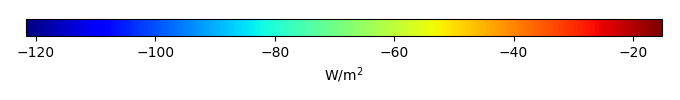MODEL MEANBIAS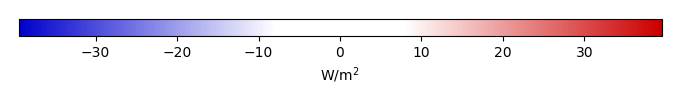BIAS SCORERMSE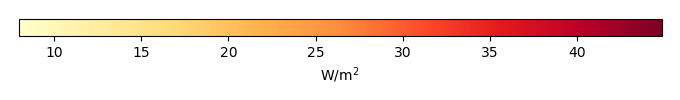RMSE SCOREBENCHMARK INTERANNUAL VARIABILITY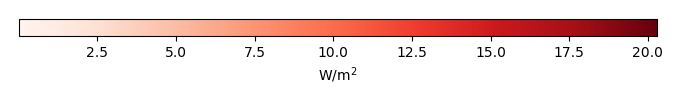MODEL INTERANNUAL VARIABILITYINTERANNUAL VARIABILITY SCOREBENCHMARK MAX MONTHMODEL MAX MONTHDIFFERENCE IN MAX MONTH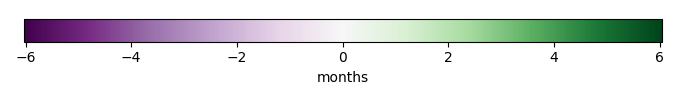SEASONAL CYCLE SCORESPATIAL TAYLOR DIAGRAMMODEL COLORS# Spatially integrated regional mean

MODEL COLORSREGIONAL MEANANNUAL CYCLEMONTHLY ANOMALYANNUAL CYCLE# All Models

BenchmarkCRUNCEPv7GSWP3v1WFDEI# Data Information

creation_date: Thu May 8 23:08:21 PDT 2014

source_file: This product is generated from monthly 1 degree CERES EBAF Radiation observations